14. WATER IS A SOURCE OF ELECTRIC ENERGY

14.1.  Common

Theoretical and experimental investigations show that water is a source of not only thermal energy and energy being available in hydrogen and oxygen, but a source of electric energy as well. The diagram of water molecule with ten electrons is given in Fig. 72. We have called this structure a charge molecule of water , , . It has turned out that there is a possibility to separate the electron, which belongs to hydrogen atom connected with the eighth electron of the hydrogen atom, from water molecule. In this case the proton of hydrogen atom will be connected with the second electron of oxygen atom, and the  water molecule will lose one electron and become a semi charged one (Fig. 100).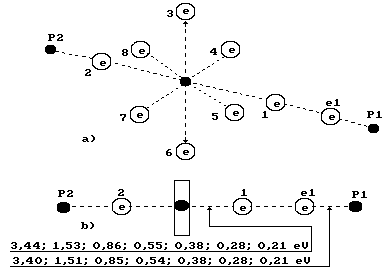Fig. 100. Diagram  of the third model of water molecule

Quantity of electricity coulombs, which is generated in one litre of water when each water molecule loses one electron, will be equal to product of Avogadro constant by quantity of moles of water molecules in one litre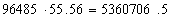Coulombs.                                                   (311)

Taking into consideration the fact that one ampere-hour is 3600 coulombs of electricity, we’ll find electrical capacity of one litre of water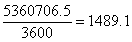Ah                                                                   (312)

Experimental investigations show that electrical potential which exceeds the potential delivered to the solution is formed in the electrolytic solution during the definite modes of plasma electrolysis of water. As a result, electric power, which exceeds electric power introduced into the solution, is generated in the electrolytic solution .

The analysis of binding energies between the electrons and the protons of the hydrogen atoms in the cluster, which consists of two water molecules (Fig. 101), shows the possibility of realization of various variants of disruption of these bonds. Under the usual conditions the bond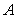is broken between the protons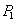and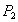, which belong to the hydrogen atoms in the water molecule. Simultaneous break of the bonds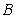and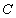is possible. In the last case, the hydrogen molecule is released. Realization of this or that variant of the bond break depends on the temperature of the environment, in which the water molecules are situated.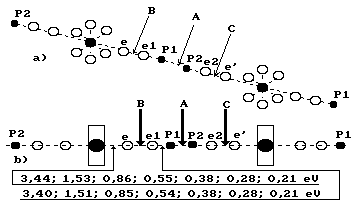Fig. 101. Diagram of the cluster, which consists of two water molecules

For example, if the water molecules are in steamy state in a cloud, realization of the breakwill lead to the formation of positively charged water molecules in the cloud. In another cloud, with another temperature, the bonds break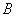oris possible as well as the formation of the negatively charge ions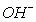in the cloud.

As realization of this or that variant of bond break depends on the temperature, we know binding energies and can model this process and use it for production of electric energy from water.

14.2.      Fuel Cell Efficiency

Fuel cells are considered to be one of the most prospective consumers of hydrogen. But efficiency of the process of the connection of hydrogen with oxygen in a fuel element as well as the formation of electric power are studied insufficiently .

The data of one of the fuel cells are given in the report . At hydrogen consumption of 2 kg per hour it generates 30 kWh of electric power. As one cubic meter of gaseous hydrogen weighs 90 g, 2 kg of liquid hydrogen contain 22.2 m3 of gaseous hydrogen. If we take into consideration that in order to produce 1 m3 of hydrogen the best industrial electrolyzers  consume 4 kWh and assume the energy value as 100%, we’ll get energy efficiency of the fuel cell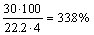.                                                                           (313)

The source of information  reports that efficiency of fuel cells of the third generation with solid electrolyte is near 50% and the use of technology of fuel cells allows to increase efficiency of electric power  up to 75%; taking into consideration heat generated by them, efficiency is increased by 90% or 95%.

The same source informs that in April, 1999, the firm “Ecostar Electric Drive Systems” (joint venture of the companies “Ford”, “Daimler-Benz “ and “Ballard Power Systems”) has been set up for elaboration of a vehicle employing fuel cells. The total amount of investments in the whole work has already reached 700 m dollars. This firm intends to begin the production of series vehicles employing fuel cells in 2004 .

Efficiency of fuel cells depends on efficiency of the  use of electric possibilities of hydrogen itself. If quantity of the electrons, which belong to the atoms of hydrogen and take part in the formation of electric power of a fuel  cell, is taken into consideration, efficiency of physical and chemical process of this cell is less than 1%. Let us make a calculation for the fuel cell, which is described in the report . This fuel cell generates 30 kW of electric power when 2 kg (22.2 m3) of liquid hydrogen is consumed per hour. As the mole of gaseous hydrogen is equal to 22.4 litres, it is necessary to consume 22222.22/22.4=992.06 moles of molecular hydrogen for the production of 30 kW of electric power .

We’d like to remind that a value equal to the product of  Avogadro number N=6.022×1023 by the electron charge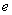= 1.602×10-19  is called Faraday constant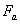. This value is measured in coulombs   (C) per mole of substance.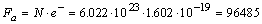C/mol.                                     (314)

If all protons of 992.06 moles of molecular hydrogen give  their electrons to electric net of the fuel cell, 992.06×2×96485=191437818.2 coulombs of electricity are formed, as a result. These are potential possibilities of 22.2 m3 of molecular hydrogen. In what way are these possibilities used by modern fuel cells?

The fuel cell being considered operates at voltage of 100 V; that’s why  when 30 kW are generated, current of 30000/100=300 amperes per hour circulates in its electric circuit. 3600 coulombs of electricity are consumed at 1 ampere/hour and 1080000.0 coulombs are consumed at 300 ampere per hour. If we assume that potential quantity  of coulombs of electricity, which 22.2 m3 of hydrogen contain (191437818.2 coulombs)is 100%, actual quantity of coulombs of electricity generated by the fuel cell is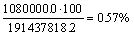(315)

These are the main reserves of efficiency improvement of the fuel cells!

Feeding of molecular hydrogen to the fuel element is the main cause of a very low (0.57%) electrical efficiency of the fuel cell. There is every reason to hope that minimal tenfold improvement of this efficiency will take place in the nearest future.

The specialists, who are busy with the investigation  of fuel cells, should pay attention to importance of the analysis of water produced in the results of its operation. We have shown that water molecules can contain both all 10 electrons (charged water) and 8 electron (discharged water). If water is pure (without impurities), there should be a difference in weight of one litre of charged water and discharged water, which can be easily found out. The greater the number of discharge molecules in water being produced after the operation of the fuel cell, the more effective the energy possibilities of hydrogen  are used.

The given calculations show that energy properties of hydrogen in fuel cells are used only by 0.6%. Tenfold increase of this index is equal to the transit to hydrogen power in all field of human activities .

The Foundations of Physchemistry of Microworld

Ó2003 Kanarev Ph. M.

Internet Version - http://book.physchemistry.innoplaza.net

<< Back to Physchemistry Book Index